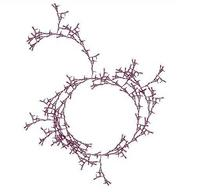University of Virginia, Department of Computer Science CS200: Computer Science, Spring 2003

Notes: Friday 14 February 2002

• Monday, 17 February (5pm): Think of one good question for Tim Koogle and email it to evans@cs.virginia.edu. Students who do not send a good question will not be permitted to attend Wednesday's class.
• Wednesday, 19 February: Problem Set 4
• Friday, 21 February: Exam 1 out (see details below).
• Monday, 24 February, 2:00PM: Exam 1 due
• Before 10 March: Read rest of GEB part I (Chapters 2-4 and 6-9, in addition to Chapters 1 and 5 you have already read).
Sorting Code
Download: sorting.ss

```(require (lib "trace.ss"))

(define (insertl f lst start)
(if (null? lst)
start
(f (car lst) (insertl f (cdr lst) start))))

;;; Evaluates to the list parameter with exactly one instance of el removed.
(define (delete lst el)
(if (null? lst) (error "Element not found!")
(if (eq? (car lst) el)
(cdr lst)
(cons (car lst) (delete (cdr lst) el)))))

(define (find-most cf lst)
(insertl
(lambda (c1 c2)
(if (cf c1 c2) c1 c2))
lst
(car lst)))

(define (bubblesort cf lst)
(if (null? lst) lst
(let ((most (find-most cf lst)))
(cons most (bubblesort cf (delete lst most))))))

(define (revintsto n)
(if (= n 0) null
(cons n (revintsto (- n 1)))))

(define (intsto n)
(reverse (revintsto n)))

(define (rand-int-list n)
(if (= n 0) null
(cons (random 10000) (rand-int-list (- n 1)))))

(define (insertel cf el lst)
(if (null? lst)
(list el)
(if (cf el (car lst))
(cons el lst)
(cons (car lst)
(insertel cf el (cdr lst))))))

(define (insertsort cf lst)
(if (null? lst)
null
(insertel cf
(car lst)
(insertsort cf (cdr lst)))))

(define (filter f lst)
(insertl
(lambda (el rest) (if (f el) (cons el rest) rest))
lst
null))

(define (quicksort cf lst)
(if (null? lst)
lst
(append
(quicksort cf
(filter (lambda (el) (cf el (car lst))) (cdr lst)))
(list (car lst))
(quicksort cf
(filter (lambda (el) (not (cf el (car lst)))) (cdr lst))))))

(define (sublist lst start end)
(if (= start 0)
(if (= end 0)
null
(cons (car lst)
(sublist (cdr lst) start (- end 1))))
(sublist (cdr lst) (- start 1) (- end 1))))

(define (first-half lst)
(sublist lst 0  (floor (/ (+ 1 (length lst)) 2))))

(define (second-half lst)
(sublist lst (floor (/ (+ 1 (length lst)) 2)) (length lst)))

(define (insertelh cf el lst)
(if (null? lst)
(list el)
(let ((fh (first-half lst))
(sh (second-half lst)))
(if (cf el (car fh))
(append (cons el fh) sh)
(if (null? sh)
(append fh (list el))
(if (cf el (car sh))
(append (insertelh cf el fh) sh)
(append fh (insertelh cf el sh))))))))

(define (insertsorth cf lst)
(if (null? lst)
null
(insertelh cf
(car lst)
(insertsorth cf (cdr lst)))))

(define (make-tree left el right)
(list left el right))

(define (get-left tree)
(first tree))

(define (get-element tree)
(second tree))

(define (get-right tree)
(third tree))

(define (insertel-tree cf el tree)
(if (null? tree)
(make-tree null el null)
(if (cf el (get-element tree))
(make-tree
(insertel-tree cf el (get-left tree))
(get-element tree)
(get-right tree))
(make-tree
(get-left tree)
(get-element tree)
(insertel-tree cf el (get-right tree))))))

(define (extract-elements tree)
(if (null? tree)
null
(append (extract-elements (get-left tree))
(cons (get-element tree)
(extract-elements (get-right tree))))))

(define (insertsort-tree cf lst)
(define (insertsort-worker cf lst)
(if (null? lst)
null
(insertel-tree cf
(car lst)
(insertsort-worker cf (cdr lst)))))
(extract-elements (insertsort-worker cf lst)))

(define testlist (rand-int-list 20))

;;; Don't worry about this procedure.  It uses some MzScheme extensions that
;;; are not part of standard Scheme.

(define (timesort sortproc len)
(let ((vals (rand-int-list len)))
(let-values ([(val utime ptime gctime) (time-apply sortproc (list < vals))])
utime)))

(define (find-ratios lst)
(if (< (length lst) 2)
null
(let ((thisone (first lst))
(nextone (second lst)))
(cons
(exact->inexact
(/ (cdr nextone)  ;; ratio of times
(cdr thisone)))
(find-ratios (cdr lst))))))

(define (testgrowth sortproc)
(let ((sizes (list 250 500 1000 2000 4000 8000 16000 32000 64000 128000)))
(find-ratios
(map (lambda (len)
(let ((time (timesort sortproc len)))
(printf "n = ~a, time = ~a~n" len time)
(cons len time)))
sizes))))

```CS 200: Computer Science Department of Computer Science University of Virginia Circle Fractal by Ramsey Arnaoot and Qi Wang cs200-staff@cs.virginia.edu Using these Materials